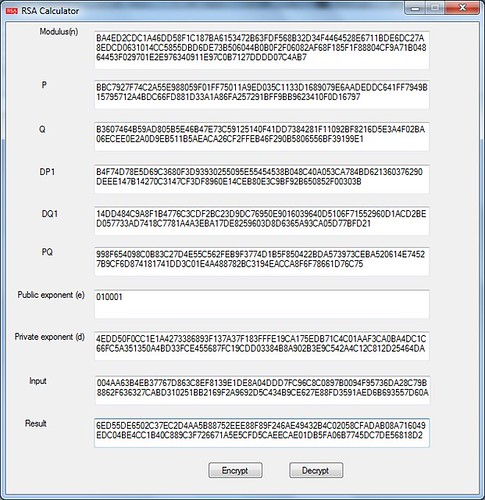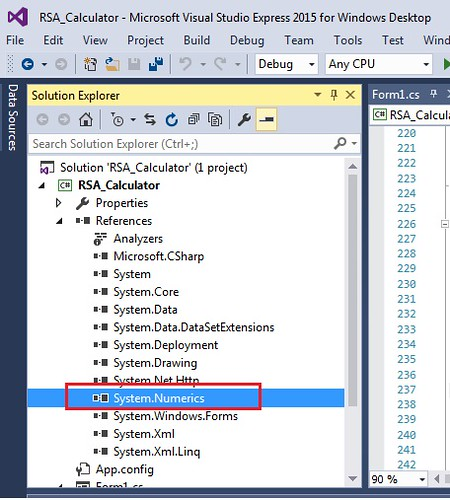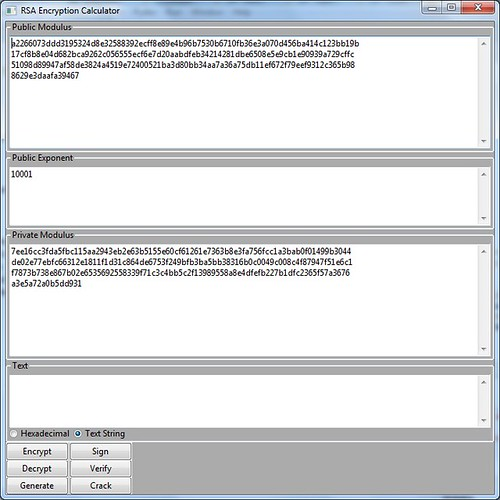# RSA algorithm and CRT in program

Explained the way to compute the RSA encryption and decryption in C sharp program, and also the way to calculate of the private key provided you know the public key, P and Q by using the extended Euclidean algorithm, reference to the Python implementation of RSA.

# Testing material with RSA

For understand the using CRT with RSA, refer to this Link. And I am not going to explain the detail as I am not quite familiar with the math in detail myself.

As long as CRT will speed up the computing of RSA, here suppose we have all the detail as Public modulus, Public Exponent, P, Q, DP1, DQ1 and PQ, we use example as below, as I have tested it, it is valid,

0x80 length of public modulus, below is the value.

83934BA65BFC8C67C9394F1AFA9D3F1025BD8DC13B566B364463A6E2F772391C722D070BA4ED2CDC1A46DD58F1C187BA6153472B63FDF568B32D34F4464528E6711BDE6DC27A8EDCD0631014CC5855DBD6DE73B506044B0B0F2F06082AF68F185F1F88804CF9A71B04864453F029701E2E976340911E97C0B7127DDDD07C4AB7

Public Exponent,below is the value

010001

0x40 – length of P, below is the value,

0x40– length of Q, below is the value,

0x40– length of DP1, below is the value,

B4F74D78E5D69C3680F3D93930255095E55454538B048C40A053CA784BD621360376290DEEE147B14270C3147CF3DF8960E14CEB80E3C9BF92B650852F00303B

0x40– length of DQ1, below is the value,

0x40– length of PQ, below is the value,

998F654098C0B83C27D4E55C562FEB9F3774D1B5F850422BDA573973CEBA520614E74527B9CF6D874181741DD3C01E4A488782BC3194EACCA8F6F78661D76C75

Private exponent(d) as below,

4EDD50F0CC1E1A4273386893F137A37F183FFFE19CA175EDB71C4C01AAF3CA0BA4DC1C66FC5A351350A4BD33FCE455687FC19CDD03384B8A902B3E9C542A4C12C812D25464DAB78815D2C8287FFA35949697B83EEEC437F6C92FA22149DDD5336C7A8A10CF165F3EED42F4FBFC362493D7D68C3641A0D36CFA015EBB6188DE81

We use below value as the plain text to encrypt:

Encrypted text as below,

Can use this link to verify the RSA encryption and decryption result.

# Verify the CRT parameters are valid

The interface of the RSA computing GUI is as below screenshot shows,Make sure you have the correct version Visual studio to import “System.Numerics” support. as below screenshot,To convert from hex string on the GUI to the byte array, can refer to this Link, by using below methods,

`public static byte[] ConvertToByteArray(string value)`

Convert all the RSA parameters to the BigIntegers,

```BigInteger P = Program.FromBigEndian(P2);
BigInteger Q = Program.FromBigEndian(Q2);
BigInteger DP = Program.FromBigEndian(DP2);
BigInteger DQ = Program.FromBigEndian(DQ2);
BigInteger InverseQ = Program.FromBigEndian(InverseQ2);
BigInteger E = Program.FromBigEndian(Exponent2);
BigInteger M = Program.FromBigEndian(Modulus2);
BigInteger M1 = BigInteger.Multiply(P, Q); // M = P*Q```

Verify M = P*Q

```if (M1.CompareTo(M) == 0)
{
//MessageBox.Show("Modulus is correct!");
}
else
{
MessageBox.Show("Modulus is not correct as M!=P*Q");
return;
}```

Calculate the private key D,

```BigInteger PMinus1 = BigInteger.Subtract(P, BigInteger.One); // P-1
BigInteger QMinus1 = BigInteger.Subtract(Q, BigInteger.One); // Q-1
BigInteger Phi = BigInteger.Multiply(PMinus1, QMinus1);
BigInteger D1 = Program.modinv(E, Phi);```

Here the Program.modinv(E, Phi) is to use the Extended Euclidean algorithm to calculate the modular inverse, the result is the private key D, E is the public key, refer to this link. The source code is as below,

```public static BigInteger modinv(BigInteger u, BigInteger v)
{
BigInteger inv, u1, u3, v1, v3, t1, t3, q;
BigInteger iter;
/* Step X1. Initialise */
u1 = 1;
u3 = u;
v1 = 0;
v3 = v;
/* Remember odd/even iterations */
iter = 1;
/* Step X2. Loop while v3 != 0 */
while (v3 != 0)
{
/* Step X3. Divide and "Subtract" */
q = u3 / v3;
t3 = u3 % v3;
t1 = u1 + q * v1;
/* Swap */
u1 = v1; v1 = t1; u3 = v3; v3 = t3;
iter = -iter;
}
/* Make sure u3 = gcd(u,v) == 1 */
if (u3 != 1)
return 0;   /* Error: No inverse exists */
/* Ensure a positive result */
if (iter < 0)
inv = v - u1;
else
inv = u1;
return inv;
}```

After calculate the private key D, display on the GUI.

Verify the DQ,

```BigInteger DQ1 = BigInteger.Remainder(D, QMinus1); // dQ = (1/e) mod (q-1)
if (DQ1.CompareTo(DQ) == 0)
{
//Console.WriteLine("  DQ Ok :)");
}
else
{
MessageBox.Show("DQ1 is not correct as dQ = (1/e) mod (q-1)");
return;
}```

Verify DP,

```BigInteger DP1 = BigInteger.Remainder(D, PMinus1); // dP = (1/e) mod (p-1)
if (DP1.CompareTo(DP) == 0)
{
//Console.WriteLine("  DP Ok :)");
}
else
{
MessageBox.Show("DP1 is not correct as dP != (1/e) mod (p-1)");
return;
}```

Verify PQ,

```//qInv = (1/q) mod p
BigInteger InverseQ1 = Program.modinv(Q, P);
if (InverseQ1.CompareTo(InverseQ) == 0)
{
//Console.WriteLine("  qInv = (1/q) mod p Ok :)");
}
else
{
MessageBox.Show("InverseQ1 is not correct as qInv = (1/q) mod p");
return;
}```

# RSA encryption and decryption

By using the C# function to encrypt and decrypt, there will be length restriction, 1024 bit length key may only encrypt 117 bytes material, refer to this link (Modulus size – 11. (11 bytes is the minimum padding possible.)). ,

```rsa.ImportParameters(Program.key);
byte[] encoded = rsa.Encrypt(input, false);
//   byte[] encoded = rsa.Decrypt(input, false);```

instead I am using the BigInteger calculation, but not sure it will work in all the case, but at least it works at my example material above,

```BigInteger c = Program.FromBigEndian(input);
BigInteger m = BigInteger.ModPow(c, D, M); //decrypt
//BigInteger c = BigInteger.ModPow(m, E, M); //encrypt if it is encryption, it will like this line..

byte[] Plaintext = ConvertToByteArray(m.ToString("X"));

if (Plaintext == 0x0)
{
byte[] newPlaintext = new byte[Plaintext.Length - 1]; //remove the first 0x00
Array.Copy(Plaintext, 1, newPlaintext, 0, Plaintext.Length - 1);
result_txt.Text = ByteArrayToHex(newPlaintext);  //remove first 0
}
else
{
result_txt.Text = ByteArrayToHex(Plaintext);
}```

# String, Array, BigInteger convert in process

As above mentioned, to convert from hex string on the GUI to the byte array, can refer to thisLink, by using below methods,

`public static byte[] ConvertToByteArray(string value)`

After converted to byte array, then converted to BigInteger, here if the highest bit is 1, needs to add 0x00 at the beginning to prevent the negative value, and we can see there is Array.Reverse() function call, it seems Microsoft BigInteger is LittleEndian,

```public static BigInteger FromBigEndian(byte[] p)
{
Array.Reverse(p);
if (p[p.Length - 1] > 127)
{
Array.Resize(ref p, p.Length + 1);  //this is to prevent the negative value.
p[p.Length - 1] = 0;
}
return new BigInteger(p);
}```

It is much easier to convert from BigInteger to the hex string and display on the GUI,

```byte[] C = ConvertToByteArray(c.ToString("X"));
result_txt.Text = ByteArrayToHex(C);```

# RSA compute in Python

There is Python program available online to calculate RSA, refer to this Link, and source code download from Here. The software screenshot is as below,Reference:

Below references are for the Python implement RSA computing,